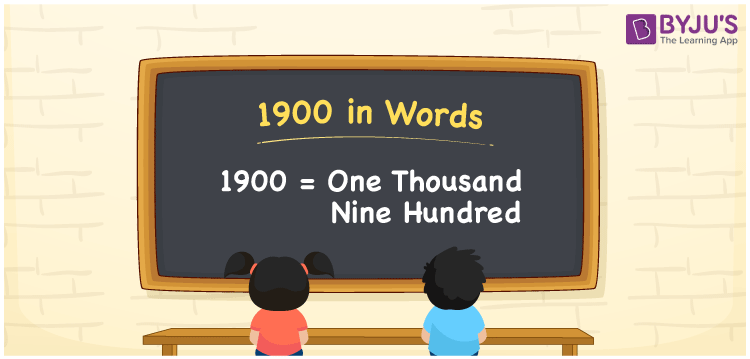# 1900 in Words

We can write 1900 in words as One Thousand Nine Hundred. It can also be represented in words as Nineteen Hundred. If you have Rs 1900 in your wallet, you can write as “I have One Thousand Nine Hundred in my wallet”. We know that we can convert any number with the help of a place value chart. Also, we know that 1900 is a cardinal number since it shows a specific quantity.

 1900 in words One Thousand Nine Hundred One Thousand Nine Hundred in numerical form 1900

## 1900 in English Words

We know that numbers in words are written using the English alphabet. Hence, in English 1900 is written as “One Thousand Nine Hundred”.## How to Write 1900 in Words?

Let us convert 1900 in words with the help of a place value chart. The number 1900 has 4 digits. Hence, let us create a chart that shows the place value up to 4 digits.

 Thousands Hundreds Tens Ones 1 9 0 0

Thus, we can write the expanded form as:

1 × Thousand + 9 × Hundred + 0 × Ten + 0 × One

= 1 × 1000 + 9 × 100 + 0 × 10 + 0 × 1

= 1000 + 900

= 1900

= One Thousand Nine Hundred

Therefore, 1900 in words is written as One Thousand Nine Hundred.

Interesting way of writing 1900 in words

1= One

19 = Nineteen

190 = One Hundred and Ninety

1900 = One Thousand Nine Hundred (Or) Nineteen Hundred

1900 is a natural number. The predecessor of 1900 is 1899 and the successor is 1901.

• 1900 in words – One Thousand Nine Hundred
• Is 1900 an odd number? – No
• Is 1900 an even number? – Yes
• Is 1900 a perfect square number? – No
• Is 1900 a perfect cube number? – No
• Is 1900 a prime number? – No
• Is 1900 a composite number? – Yes

## Frequently Asked Questions on 1900 in Words

Q1

### How to write 1900 in words?

1900 in words is written as One Thousand Nine Hundred.
Q2

### Calculate the value of 1520 + 380 and write the answer in words.

On simplifying 1520 + 380 we get 1900. 1900 in words is written as One Thousand Nine Hundred.
Q3

### 1900 is a composite number. True or False.

True. 1900 is a composite number.